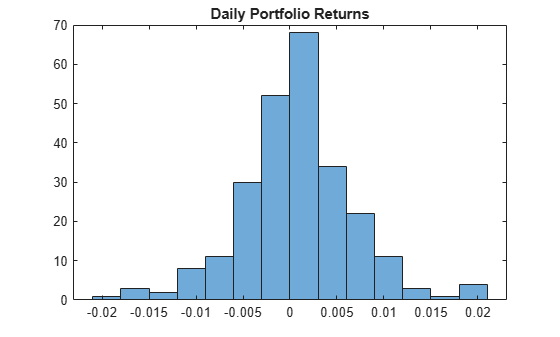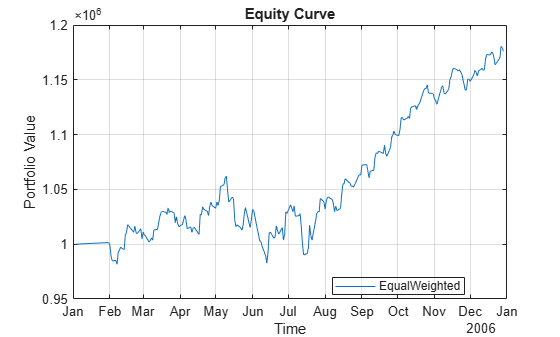# backtestEngine

Create `backtestEngine` object to backtest strategies and analyze results

## Description

Create a `backtestEngine` to run a backtest of portfolio investment strategies on historical data.

Use this workflow to develop and run a backtest:

1. Define the strategy logic using a `backtestStrategy` object to specify how a strategy rebalances a portfolio of assets.

2. Use `backtestEngine` to create a `backtestEngine` object that specifies parameters of the backtest.

3. Use `runBacktest` to run the backtest against historical asset price data and, optionally, trading signal data.

4. Use `equityCurve` to plot the equity curves of each strategy.

5. Use `summary` to summarize the backtest results in a table format.

For more detailed information on this workflow, see Backtest Investment Strategies Using Financial Toolbox™.

## Creation

### Syntax

``backtester = backtestEngine(strategies)``
``backtester = backtestEngine(___,Name,Value)``

### Description

example

````backtester = backtestEngine(strategies)` creates a `backtestEngine` object. Use the `backtestEngine` object to backtest the portfolio trading strategies defined in the `backtestStrategy` objects.```

example

````backtester = backtestEngine(___,Name,Value)` sets properties using name-value pair arguments and any of the arguments in the previous syntax. You can specify multiple name-value pair arguments. For example, ```backtester = backtestEngine(strategies,'RiskFreeRate',0.02,'InitialPortfolioValue',1000,'RatesConvention',"Annualized",'Basis',2)```.```

### Input Arguments

expand all

Backtest strategies, specified as a vector of `backtestStrategy` objects. Each `backtestStrategy` object defines a portfolio trading strategy.

Data Types: `object`

Name-Value Arguments

Specify optional pairs of arguments as `Name1=Value1,...,NameN=ValueN`, where `Name` is the argument name and `Value` is the corresponding value. Name-value arguments must appear after other arguments, but the order of the pairs does not matter.

Before R2021a, use commas to separate each name and value, and enclose `Name` in quotes.

Example: ```backtester = backtestEngine(strategies,'RiskFreeRate',0.02,'InitialPortfolioValue',1000,'RatesConvention',"Annualized",'Basis',2)```

Risk free rate, specified as the comma-separated pair consisting of `'RiskFreeRate'` and a scalar numeric or a one-column timetable.

Note

If you specify a timetable:

• The dates in the specified `timetable` must include the start and end dates of the backtest.

• The series of dates in the specified timetable between the start and end dates (inclusive) must correspond exactly to the corresponding series of dates in the `assetPrices` timetable.

If `RatesConvention` is `"Annualized"`, then `RiskFreeRate` specifies an annualized rate.

If `RatesConvention` is `"PerStep"`, then the `RiskFreeRate` is a decimal percentage and represents the risk free rate for one time step in the backtest. For example, if the backtest uses daily asset price data, then the `RiskFreeRate` value must be the daily rate of return for cash.

Data Types: `double` | `timetable`

Cash borrowing rate, specified as the comma-separated pair consisting of `'CashBorrowRate'` and a scalar numeric or a one-column timetable.

Note

If you specify a timetable:

• The dates in the specified `timetable` must include the start and end dates of the backtest.

• The series of dates in the specified timetable between the start and end dates (inclusive) must correspond exactly to the corresponding series of dates in the `assetPrices` timetable.

The `CashBorrowRate` specifies the rate of interest accrual on negative cash balances (margin) during the backtest.

If `RatesConvention` is `"Annualized"`, then `CashBorrowRate` specifies an annualized rate.

If `RatesConvention` is `"PerStep"`, then the `CashBorrowRate` value is a decimal percentage and represents the interest accrual rate for one time step in the backtest. For example, if the backtest is using daily asset price data, then the `CashBorrowRate` value must be the daily interest rate for negative cash balances.

Data Types: `double` | `timetable`

Initial portfolio value, specified as the comma-separated pair consisting of `'InitialPortfolioValue'` and a scalar numeric.

Data Types: `double`

Defines how backtest engine uses `RiskFreeRate` and `CashBorrowRate` to compute interest, specified as the comma-separated pair consisting of `'RatesConvention'` and a character vector or string.

• `'Annualized'` — The rates are treated as annualized rates and the backtest engine computes incremental interest based on the day count convention specified in the `Basis` property. This is the default.

• `'PerStep'` — The rates are treated as per-step rates and the backtest engine computes interest at the provided rates at each step of the backtest.

Data Types: `char` | `string`

Date handling behavior for rebalance dates that are missing from asset prices timetable, specified as the comma-separated pair consisting of `'DateAdjustment'` and a character vector or string.

• `'Previous'` — For each rebalance date in the rebalance schedule, the rebalance occurs on the nearest date in the asset timetable that occurs on or before the requested rebalance date. This is the default.

• `'Next'` — Move to the next date.

• `'None'` — Dates are not adjusted and the backtest engine errors when encountering a rebalance date that does not appear in the asset prices timetable.

Data Types: `char` | `string`

Defines the day-count convention when computing interest at the `RiskFreeRate` or `CashBorrowRate`, specified as the comma-separated pair consisting of `'Basis'` and a scalar integer using a supported value:

• 0 = actual/actual

• 1 = 30/360 (SIA)

• 2 = actual/360

• 3 = actual/365

• 4 = 30/360 (PSA)

• 5 = 30/360 (ISDA)

• 6 = 30/360 (European)

• 7 = actual/365 (Japanese)

• 8 = actual/actual (ICMA)

• 9 = actual/360 (ICMA)

• 10 = actual/365 (ICMA)

• 11 = 30/360E (ICMA)

• 12 = actual/365 (ISDA)

• 13 = BUS/252

Note

`Basis` is only used when the `RatesConvention` property is set to `"Annualized"`. If the `RatesConvention` is `"PerStep"`, and `Basis` is set, `backtestEngine` ignores the `Basis` value.

Data Types: `double`

## Properties

expand all

Backtest strategies, specified as a vector of `backtestStrategy` objects.

Data Types: `object`

Risk free rate, specified as a scalar numeric or timetable.

Data Types: `double`

Cash borrowing rate, specified as a scalar numeric or timetable.

Data Types: `double`

Initial portfolio value, specified as a scalar numeric.

Data Types: `double`

Use annualized rates for `RiskFreeRate` and `CashBorrowRate`, specified as a scalar logical.

Data Types: `logical`

Date handling behavior for rebalance dates that are missing from asset prices timetable, specified as a string.

Data Types: `char` | `string`

Day-count of annualized rates for `RiskFreeRate` and `CashBorrowRate`, specified a scalar integer.

Data Types: `double`

This property is read-only.

Number of assets in the portfolio universe, a numeric. `NumAssets` is derived from the timetable of adjusted prices passed to `runBacktest`. `NumAssets` is empty until you run the backtest using the `runBacktest` function.

Data Types: `double`

This property is read-only.

Strategy returns, a `NumTimeSteps`-by-`NumStrategies` timetable of strategy returns. Returns are per time step. For example, if you use daily prices with `runBacktest`, then `Returns` is the daily strategy returns. `Returns` is empty until you run the backtest using the `runBacktest` function.

Data Types: `timetable`

This property is read-only.

Asset positions for each strategy, a structure containing a `NumTimeSteps`-by-`NumAssets` timetable of asset positions for each strategy. For example, if you use daily prices in the `runBacktest`, then the `Positions` structure holds timetables containing the daily asset positions. `Positions` is empty until you run the backtest using the `runBacktest` function.

Data Types: `struct`

This property is read-only.

Strategy turnover, a `NumTimeSteps`-by-`NumStrategies` timetable. `Turnover` is empty until you run the backtest using the `runBacktest` function.

Data Types: `timetable`

This property is read-only.

Transaction costs for the asset purchases of each strategy, a `NumTimeSteps`-by-`NumStrategies` timetable. `BuyCost` is empty until you run the backtest using the `runBacktest` function.

Data Types: `timetable`

This property is read-only.

Transaction costs for the asset sales of each strategy, a `NumTimeSteps`-by-`NumStrategies` timetable. `SellCost` is empty until you run the backtest using the `runBacktest` function.

Data Types: `timetable`

This property is read-only.

Paid fees for management and performance fees, a struct containing a timetable for each strategy which holds all the fees paid by the strategy. The `Fees` timetable contains an entry for each date where at least one fee was paid. Each column holds the amount paid for a particular type of fee. If no fees are paid, then the `Fees` timetable is empty.

For more information on management and performance fees defined using a `backtestStrategy` object, see Management Fees, Performance Fees, and Performance Hurdle.

Data Types: `timetable`

## Object Functions

 `runBacktest` Run backtest on one or more strategies `summary` Generate summary table of backtest results `equityCurve` Plot equity curves of strategies

## Examples

collapse all

Use a backtesting engine in MATLAB® to run a backtest on an investment strategy over a time series of market data. You can define a backtesting engine by using `backtestEngine` object. A `backtestEngine` object sets properties of the backtesting environment, such as the risk-free rate, and holds the results of the backtest. In this example, you can create a backtesting engine to run a simple backtest and examine the results.

Create Strategy

Define an investment strategy by using the `backtestStrategy` function. This example builds a simple equal-weighted investment strategy that invests equally across all assets. For more information on creating backtest strategies, see `backtestStrategy`.

```% The rebalance function is simple enough that you can use an anonymous function equalWeightRebalanceFcn = @(current_weights,~) ones(size(current_weights)) / numel(current_weights); % Create the strategy strategy = backtestStrategy("EqualWeighted",equalWeightRebalanceFcn,... 'RebalanceFrequency',20,... 'TransactionCosts',[0.0025 0.005],... 'LookbackWindow',0)```
```strategy = backtestStrategy with properties: Name: "EqualWeighted" RebalanceFcn: [function_handle] RebalanceFrequency: 20 TransactionCosts: [0.0025 0.0050] LookbackWindow: 0 InitialWeights: [1x0 double] ManagementFee: 0 ManagementFeeSchedule: 1y PerformanceFee: 0 PerformanceFeeSchedule: 1y PerformanceHurdle: 0 ```

Set Backtesting Engine Properties

The backtesting engine has several properties that you set by using parameters to the `backtestEngine` function.

Risk-Free Rate

The `RiskFreeRate` property holds the interest rate earned for uninvested capital (that is, cash). When the sum of portfolio weights is below 1, the remaining capital is invested in cash and earns the risk-free rate. The risk-free rate and the cash-borrow rate can be defined in annualized terms or as explicit "per-time-step" interest rates. The `RatesConvention` property is used to specify how the `backtestEngine` interprets the two rates (the default interpretation is "Annualized"). For this example, set the risk-free rate to 2% annualized.

```% 2% annualized risk-free rate riskFreeRate = 0.02;```

Cash Borrow Rate

The `CashBorrowRate` property sets the interest accrual rate applied to negative cash balances. If at any time the portfolio weights sum to a value greater than 1, then the cash position is negative by the amount in excess of 1. This behavior of portfolio weights is analogous to borrowing capital on margin to invest with leverage. Like the `RiskFreeRate` property, the `CashBorrowRate` property can either be annualized or per-time-step depending on the value of the `RatesConvention` property.

```% 6% annualized margin interest rate cashBorrowRate = 0.06;```

Initial Portfolio Value

The `InitialPortfolioValue` property sets the value of the portfolio at the start of the backtest for all strategies. The default is \$10,000.

```% Start backtest with \$1M initPortfolioValue = 1000000;```

Create Backtest Engine

Using the prepared properties, create the backtesting engine using the `backtestEngine` function.

```% The backtesting engine takes an array of backtestStrategy objects as the first argument backtester = backtestEngine(strategy,... 'RiskFreeRate',riskFreeRate,... 'CashBorrowRate',cashBorrowRate,... 'InitialPortfolioValue',initPortfolioValue)```
```backtester = backtestEngine with properties: Strategies: [1x1 backtestStrategy] RiskFreeRate: 0.0200 CashBorrowRate: 0.0600 RatesConvention: "Annualized" Basis: 0 InitialPortfolioValue: 1000000 DateAdjustment: "Previous" NumAssets: [] Returns: [] Positions: [] Turnover: [] BuyCost: [] SellCost: [] Fees: [] ```

Several additional properties of the backtesting engine are initialized to empty. The backtesting engine populates these properties, which contain the results of the backtest, upon completion of the backtest.

Load Data and Run Backtest

Run the backtest over daily price data from the 30 component stocks of the DJIA.

```% Read table of daily adjusted close prices for 2006 DJIA stocks T = readtable('dowPortfolio.xlsx'); % Remove the DJI index column and convert to timetable pricesTT = table2timetable(T(:,[1 3:end]),'RowTimes','Dates');```

Run the backtest using the `runBacktest` function.

`backtester = runBacktest(backtester,pricesTT)`
```backtester = backtestEngine with properties: Strategies: [1x1 backtestStrategy] RiskFreeRate: 0.0200 CashBorrowRate: 0.0600 RatesConvention: "Annualized" Basis: 0 InitialPortfolioValue: 1000000 DateAdjustment: "Previous" NumAssets: 30 Returns: [250x1 timetable] Positions: [1x1 struct] Turnover: [250x1 timetable] BuyCost: [250x1 timetable] SellCost: [250x1 timetable] Fees: [1x1 struct] ```

Examine Results

The backtesting engine populates the read-only properties of the `backtestEngine` object with the backtest results. Daily values for portfolio returns, asset positions, turnover, transaction costs, and fees are available to examine.

Examine the daily returns.

```% Generate a histogram of daily portfolio returns histogram(backtester.Returns{:,1}) title('Daily Portfolio Returns')```Use `equityCurve` to plot the equity curve for the simple equal-weighted investment strategy.

`equityCurve(backtester)`## Version History

Introduced in R2020b

expand all